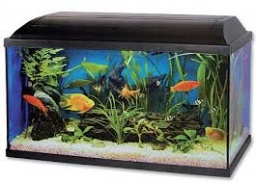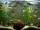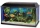# The aquarium

The aquarium is 120 cm long, 70 cm wide and 96 cm high. How high does the water reach if they filled 168l of water into the aquarium?

h =  20 cm

### Step-by-step explanation:Did you find an error or inaccuracy? Feel free to write us. Thank you!Tips to related online calculators
Do you want to convert length units?
Do you know the volume and unit volume, and want to convert volume units?

## Related math problems and questions:

• The cuboidThe cuboid is full to the brim with water. The external dimensions are 95 cm, 120 cm, and 60 cm. The thickness of all walls and the bottom is 5 cm. How many liters of water fit into the cuboid?
• Aquarium heightHow high does the water in the aquarium reach, if there are 36 liters of water in it? The length of the aquarium is 60 cm and the width is 4 dm.
• TrayWhat height reach water level in the tray shaped a cuboid, if it is 420 liters of water and bottom dimensions are 120 cm and 70 cm.
• Aquarium 6How high is the water level in the aquarium with a rectangular base 40cm and 50cm if it is filled 0,65hl of water?
• Water in aquariumThe aquarium cuboid shape with a length of 25 cm and a width of 30 cm is 9 liters of water. Calculate the areas which are wetted with water.
• Water tank300hl of water was filled into the tank 12 m long and 6 m wide. How high does it reach?
• SnailsHow many liters of water will fit in an aquarium with bottom dimensions of 30 cm and 25 cm and a height of 60 cm, if we pour water up to a height of 58 cm? How many most snails can we keep in an aquarium, if we know that snails need 600 cm ^ 3 of water fo
• Cuboid aquariumCuboid 25 times 30 cm. How long is third side if cuboid contains 30 liters of water?
• AquariumThe box-shaped aquarium is 40 cm high; the bottom has dimensions of 70 cm and 50 cm. Simon wanted to create an exciting environment for the fish, so he fixed three pillars to the bottom. They all have the shape of a cuboid with a square base. The base edg
• Four prismsQuestion No. 1: The prism has the dimensions a = 2.5 cm, b = 100 mm, c = 12 cm. What is its volume? a) 3000 cm2 b) 300 cm2 c) 3000 cm3 d) 300 cm3 Question No.2: The prism base is a rhombus with a side length of 30 cm and a height of 27 cm. The height of t
• Cube-shaped containerThe cube-shaped container has a height of 52 cm and a square base. The container was filled to the brim with water, then we immersed a metal cube in it, which caused 2.7 l of water to flow out of the container. After removing the cube from the water, the
• AquariumFind how many dm2 of glass we need to make a block-shaped aquarium (the top is not covered) if the dimensions of the aquarium are to be: width 50 cm, length 120 cm, and height 8.5 dm.
• AquariumTry to estimate the weight of the water in an aquarium 50cm long 30cm wide, when poured to a height of 25cm. Calculates the weight of the aquarium's water.
• Water tankWhat is the height of the cuboid-shaped tank with the bottom dimensions of 80 cm and 50 cm if the 480 liters of water reach 10 cm below the top?
• Swimming pool 4The pool shaped cuboid measuring 12.5 m × 640 cm at the bottom is 960hl water. To what height in meters reaches the water level?
• Volume per timeHow long does fill take for a pump with a volume flow of 200 l per minute fill a cube-shaped tank up to 75% of its height if the length of the cube edge is 4 m?
• AquariumI have an aquarium that is 100 cm long and 40 cm wide and 40 cm in height. We fill it with water. How much will it weigh?